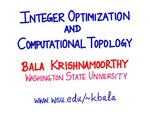Systems Science Friday Noon Seminar Series

4-22-2011

Abstract

We present recently discovered connections between integer optimization, or integer programming (IP), and homology. Under reasonable assumptions, these results lead to efficient solutions of several otherwise hard-to-solve problems from computational topology and geometric analysis. The main result equates the total unimodularity of the boundary matrix of a simplicial complex to an algebraic topological condition on the complex (absence of relative torsion), which is often satisfied in real-life applications . When the boundary matrix is totally unimodular, the problem of finding the shortest chain homologous under Z (ring of integers) to a given chain, which is inherently an integer program, can be solved in polynomial time as a linear program. This result is surprising in the backdrop of a previous result, which showed the problem to be NP-hard when the homology is defined over the popularly used field of Z2, consisting of integers 0 and 1. This problem finds applications in several domains including coverage verification in sensor networks and characterizing tunnels in biomolecules. We also present new results on computing the flat norm of currents in the setting of simplicial complexes. Flat norm decomposition is a classical technique from geometric measure theory, and has been applied for several image analysis tasks. Our approach allows one to use flat norm computations in arbitrarily large dimensions - for instance, to denoise high dimensional datasets.

Biographical Information

Bala Krishnamoorthy is an assistant professor in the Department of Mathematics at Washington State University. After earning his Bachelors degree from Indian Institute of Technology, Madras, he obtained a PhD in Operations Research from University of North Carolina at Chapel Hill. His areas of research interest are diverse. Bala tackles theoretical and computational problems from the areas of algebraic topology and discrete optimization (integer programming and combinatorial optimization). He also works on several application areas, including computational approaches to protein structure and function, and models for human neck anatomy and physiology. He collaborates with mathematicians, computer scientists, biochemists, and bioengineers.

Subjects

Integer programming, Homology theory, Algebraic topology, System theory, Computational complexity

Disciplines

Algebraic Geometry | Geometry and Topology

Persistent Identifier

https://archives.pdx.edu/ds/psu/31165COinS